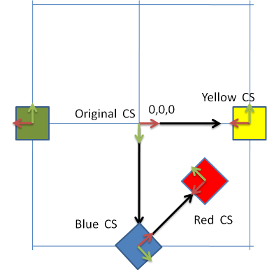Basic algebra

How to: Translate - Rotate - Scale

Basic algebra for users. When I hear about Matrixes and Vectors I am not scared, but I know that troubles are near, because vector algebra can cause me headache. What I usualy need is a basic cookbook with most basic user recipes, "How to do it," and that is what I want to offer here.

The most basic space is simple: one origin (point 0, 0, 0), one x-axis (vector 1, 0, 0), y-axis and z-axis. But whenever we place an object in such space, we are not necessarily aware that we also created a subspace - the object space. From this moment, we can place other objects not only in the main space but also in this subspace, using the method addChild, or addParent. Each space is defined by its coordination system, for short CS:

Object3D cube = Primitives.getCube(1f);
Object3D sphere = Primitives.getSphere(1f);
cube.translate(0f,5f,0f);
sphere.translate(5f,0f,0f);

The cube in this example is defined in the main CS translated by 5 in the Y direction, but because the sphere is the cube's child, it is defined in the cubes CS, so it is moved by 5 in X direction, but not from the origin of the main CS, but from the origin of the cubes CS.

Similar examples can be found also for rotation and scale and when all those features combine, it can be hard to track them.

Scale

Probably the most simple transformation is scale. This transformation changes size of the object from the selected point called pivot. There are three catches using scale:

1) Each object has its own base size coming from the objects mesh.

Solution: The base mesh size can be found, look at the help of the method Mesh.getBoundingBox()

float[] bbox = object.getMesh().getBoundingBox();

2) The objects pivot is calculated point which means that the basic scale is not well predictable

Solution: The pivot is calculated during the Object3D.build() command. After this line, the pivot can be read and edited

object.build();
SimpleVector pivot = object.getRotationPivot();
object.setRotationPivot(newPivot);

3) The object is also scaled by all the scales of its parents, so if the size of the mesh is 1 and the objects scale is 1 that does not mean that the size will be 1, because the object can have a parent with a scale not-equal 1.

Solution: Calculate the size from the scale of all objects parents.

public static SimpleVector getSize(Object3D object){
float[] bbox = object.getMesh().getBoundingBox();
float s = object.getScale();
Object3D[] par;
par = object.getParents();
while(par.length>0){
s = s*par.getScale();
par = par.getParents();
}
SimpleVector out = new SimpleVector((bbox-bbox)*s,(bbox-bbox)*s,(bbox-bbox)*s);
return out;
}

Rotate

When rotating an object there is no problem, if the object or its parents are translated or scaled, the achieved rotation is always the same. BUT the rotation and the rotation pivot are different story. Whenever the objects parents are rotated, their CSs are rotated as well so the rotation is by different axis than you may think. Whenever the objects or its parents pivot is translated, the final orientation will be the same, but the placement of the rotated object may be totally off. <pos> red.addParent(blue); green.setRotationPivot(new SimpleVector(-5f,0f,0f)); blue.translate(0f,5f,0f); ... green.translate(5f,0f,0f); green.rotateZ((float)Math.PI); // The same way also the yellow and the red </pos>On the example, the yellow, green and red cubes are translated by same vector 5,0,0 and rotated by the same angle rotateZ(PI), but:

1) The red cube is in the CS of the blue cube

2) The green cube has translated pivot by vector -5,0,0